## A reheat Rankine cycle operates with water as the working fluid. Steam enters the first turbine at 8 MPa and 450°C and exits at 0.8 MPa. It

Question

A reheat Rankine cycle operates with water as the working fluid. Steam enters the first turbine at 8 MPa and 450°C and exits at 0.8 MPa. It is then reheated to 400°C before entering the second turbine, where it exits at 10 kPa. If the amount of work into the pump is 8.04 kJ/kg and the net work per cycle produced is 1410.25 kJ/kg, determine the thermal efficiency of the cycle

in progress 0
3 weeks 2021-08-23T23:25:10+00:00 1 Answers 1 views 0

The thermal efficiency,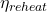, of the Rankine cycle with reheat is 36.81%

Explanation:

p₁ = 8 MPa = 80 Bars

T₁ = 450°C = 723.15 K

From steam tables, we have;

v₁ = 0.0381970 m³/kg

h₁ = 3273.23 kJ/kg

s₁ = 6.5577 kJ/(kg·K) = s₂

The p₂ = 0.8 MPa

T₂ = Saturation temperature at 0.8 MPa = 170.414°C = 443.564 K

h₂ = 2768.30 kJ/kg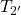= 400°C = 673.15 K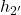= at 400°C and 0.8 MPa = 3480.6 kJ/kg

p₃ = 10 kPa = 0.1 Bar

T₃ = Saturation temperature at 10 kPa = 45.805 °C = 318.955 K

h₃ = 2583.89 kJ/kg

h₄ =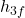= 191.812 kJ/kg

The thermal efficiency,, of a Rankine cycle with reheat is given as follows;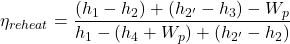Therefore, we have;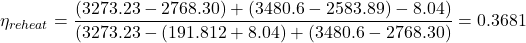Which in percentage is 36.81%.